# VBA Operators

Operators are code elements used to perform operations.

In the equation 3 + 4, the + sign is the operator.

## VBA Arithmetic Operators

Arithmetic operators are used only for numerical types, performing mathematical operations.

The appropriate data types for these operators are:

• Byte
• Integer
• Long
• Single
• Double
• Currency
• Decimal
Operator Name Example Result
+ Sum
Sub sum()

MsgBox 3 + 4

End Sub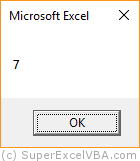7
- Subtraction
Sub subtraction()

MsgBox 7 - 2

End Sub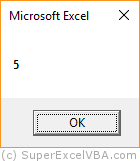5
* Multiplication
Sub multiplica()

MsgBox 4 * 2

End Sub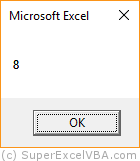8
/ Division
Sub division()

MsgBox 6 / 3

End Sub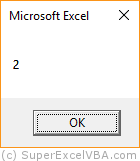2
Mod Modulus (Remainder)
Sub remainder()

MsgBox 6 Mod 5

End Sub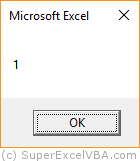1
^ Exponentiation
Sub exponentiat()

MsgBox 2 ^ 4

End Sub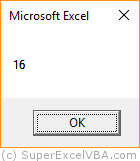16

### Calculation Order

Excel sets as calculation priority to perform first:

1. Multiplications, Divisions e Exponentiations
2. Modulus (operator that returns the remainder of a division)
3. Sums and Subtractions

The arithmetic operations are always performed from left to right ($\rightarrow$), following the calculation priority.

    MsgBox 3+6*2/3In this case 3+6*2/3, Excel performed first the 6*2, followed by the (12)/3 and only then the 3+(4), resulting in 7. This calculation can be represented mathematically as follows: $3+{(6 \times 2) \over 3}$.

Note that assigning values to variables occurs from right to left while the execution of operations occurs from left to right.

## Concatenation Operators

Excel uses the & "ampersand sign" as the main concatenation operator.

The appropriate data type for these operator is:

• String

When you concatenate one text data with another, they become a single text containing both data.

Sub Concatenateda()

Text = "Hello VBA World. " & "This tutorial is amazing!"
MsgBox Text

End Sub


The result in the concatenation above will be "Hello VBA World. This tutorial is awesome!". Notice that the space left at the end of "Hello World VBA. " Leaves the adequate spacing between sentences.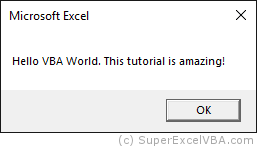Numbers concatenated with the & operator are considered text.

Sub ConcatenatNumbers()
MsgBox 13 & 25
End Sub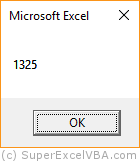The plus sign (+) can also be used to concatenate text. But avoid this practice because VBA can perform an unwanted sum with numeric variables.

You can concatenate multiple texts at the same time.

    Nome = "John"
Recompensa = "Car"
MsgBox "The winner is " & Nome & "." & " And he won a " & Recompensa & "."


Double quotation marks are not required for variables, but they are necessary for expressions.

Insert spaces between concatenated expressions so that Excel creates the phrase with the correct spacing between words.

## Comparison Operators

Comparison Operators are used to compare two expressions. The result will always be True or False.

Operations with comparison operators result in True or False, similarly to Boolean variables. Therefore, they may also be subjected to display translation.

The appropriate data types for these operator are:

• Byte
• Integer
• Long
• Single
• Double
• Currency
• Decimal
• String
• Date
• Boolean

The examples of comparison operators below will be given by comparing Integers:

Operator Description Example Result
= Is equal to
Sub equals()

MsgBox 3 = 4

End Sub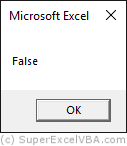Falso
> Is greater than
Sub greater()

MsgBox 7 > 2

End Sub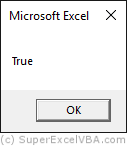< Is less than
Sub less()

MsgBox 4 < 2

End SubFalso
>= Is greater than or equal to
Sub greatequal()

MsgBox 6 >= 3

End Sub<= Is less than or equal to
Sub lessequal()

MsgBox 6 <= 5

End SubFalso
<> Is not equal to
Sub inequality()

MsgBox 2 <> 4

End SubThe equal sign (=) is used to assign a value to a variable except when there is a function before it:

  a=3 'Assigns
Msgbox a=4 'Compares


If you compare different data types (E.g. numeric with text), you may get an error:

Sub DifferentData()
MsgBox "Some text" = 7
End Sub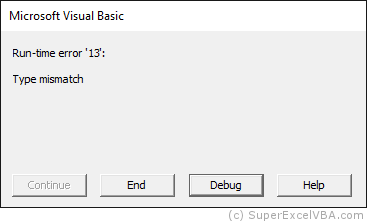You can use the =, <>, <, <=, > and >= operators to compare text.

The operator = and the operator <> will have an intuitive behavior, resulting in True or False according to the equality of left and right texts.

Sub CompareTextEgual()
MsgBox "first" = "second"
'Returns False because the texts are different
End Sub

Operators <, <=, > e >= work according to the alphabetical order, comparing letter to letter of each side, from left to right.

Sub CompareTextLess()
MsgBox "first" < "second"
'Returns True because "f" comes before "s" in the alphabetical order
End Sub

Excel evaluates the alphabetical position of the first character of each of the two texts. If both characters are the same, it compares the second and so on.

If there is an extra character on one side, the comparison will be as if the missing character was "", equivalent to 0.

E.g. MsgBox "first" > "firs" $\rightarrow$ True, because "t" > ""

Excel differs from uppercase to lowercase.

E.g. MsgBox "VBA" > "vba" $\rightarrow$ False